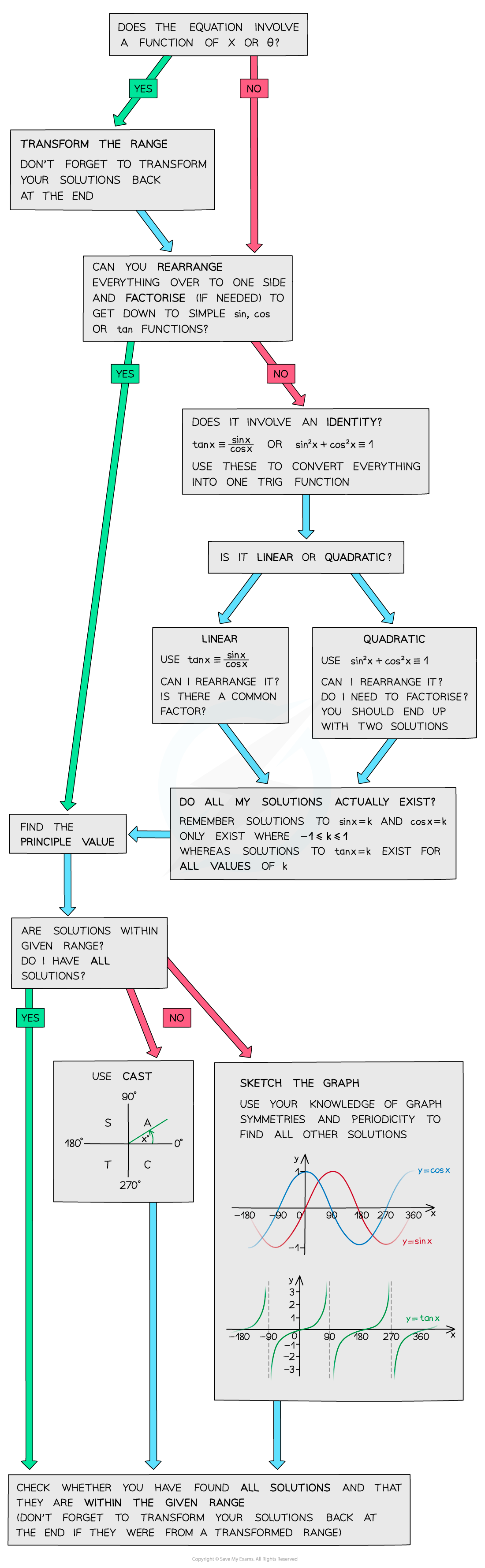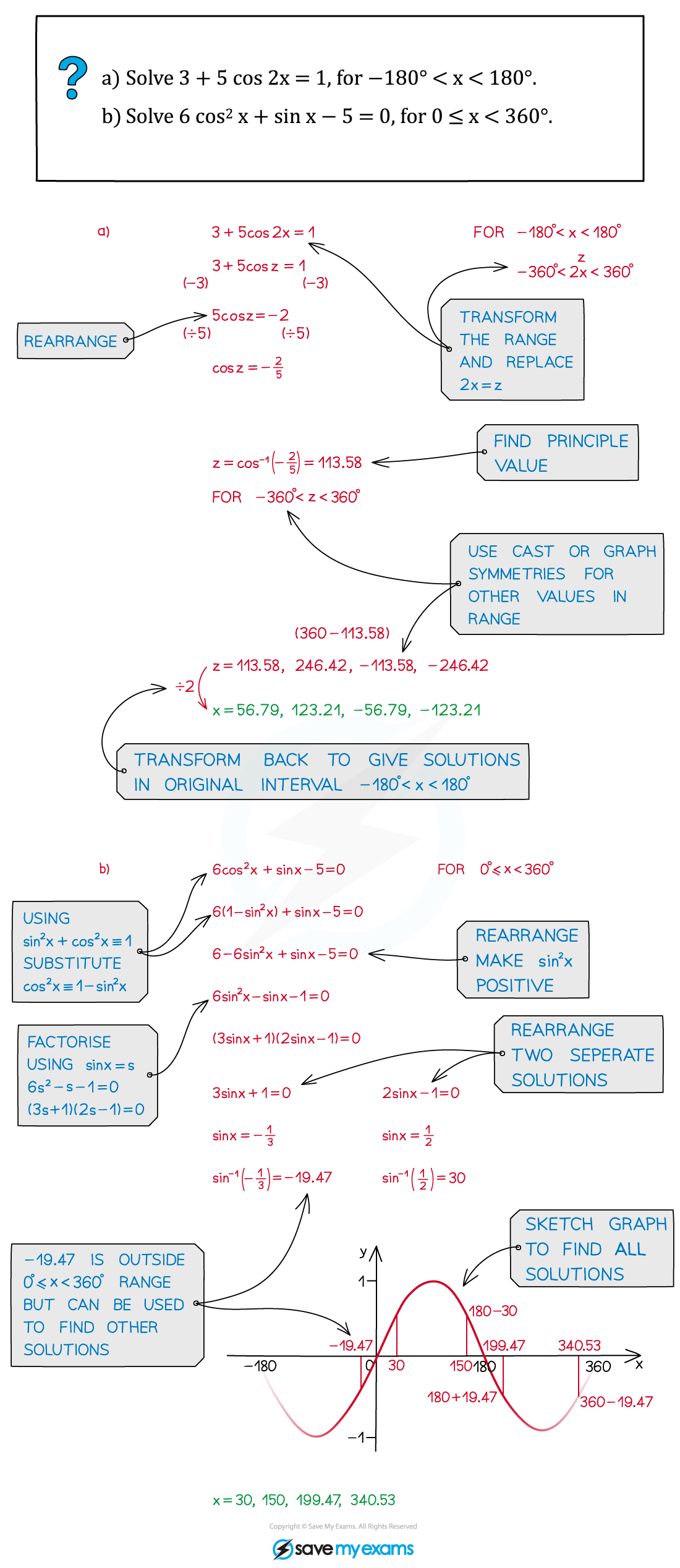# AQA A Level Maths: Pure复习笔记5.3.4 Strategy for Trigonometric Equations

### Strategy for Trigonometric Equations

#### How to approach solving trig equations

• You can solve trig equations in a variety of different ways
• Sketching a graph (see Graphs of Trigonometric Functions)
• Using trigonometric identities (see Trigonometry – Simple Identities)
• Using the CAST diagram (see Linear Trigonometric Equations)
• Factorising quadratic trig equations (see Quadratic Trigonometric Equations)
• If you’re having trouble solving a trig equation, this flowchart might help:#### Exam Tip

• Don’t forget to check the function range and ensure you have included all possible solutions.
• If the question involves a function of x or θ, make sure you transform the range first (and ensure you transform your solutions back again at the end!).

#### Worked Example